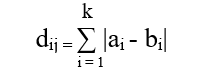Related Articles

# Python | Calculate City Block Distance

• Last Updated : 11 May, 2020

City block distance is generally calculated between 2-coordinates of a paired object. It is the summation of absolute difference between 2-coordinates. The city block distance of 2-points a and b with k dimension is mathematically calculated using below formula:In this article two solution are explained for this problem – one with the Python code and the other one with the use of a predefined method.

Examples:

Input:

array1 = [1, 2, 13, 5]
array2 = [1, 27, 3, 4]
Output:
The CityBlock distance between 2 array is: 36

Input:

a = [34, 44, 89, 81, 67, 49, 33, 59]
b = [98, 34, 23, 12, 233, 23, 44]
Output:
The CityBlock distance between 2 array is: 412

Code: Python scratch code to calculate the distance using the above formula.

 `import` `numpy as np`` ` `def` `cityblock_distance(A, B):`` ` `    ``result ``=` `np.``sum``([``abs``(a ``-` `b) ``for` `(a, b) ``in` `zip``(A, B)])``    ``return` `result`` ` `if` `__name__``=``=` `"__main__"``:`` ` `    ``array1 ``=` `[``1``, ``2``, ``13``, ``5``]``    ``array2 ``=` `[``1``, ``27``, ``3``, ``4``]`` ` `    ``result ``=` `cityblock_distance(array1, array2)`` ` `    ``print``(``"The CityBlock distance between 2 arrays is:"``, result)`

Output:

```The CityBlock distance between 2 arrays is: 36
```

There is no restriction for the same size of arrays. So, it’s easy to calculate City Block distance between 2-coordinates using above formula but we can calculate the same using predefined method for arrays having 2-dimension or more.

Code: Python code using the predefined method to calculate the distance using the above formula.

 `# Import necessary package``import` `scipy.spatial.distance as d`` ` `def` `CityBlock_distance(A, B):`` ` `    ``result ``=` `d.cdist(A, B, ``'cityblock'``)``    ``return` `result`` ` `if` `__name__``=``=` `"__main__"``:`` ` `    ``mat1 ``=` `[[``1``, ``2``, ``13``, ``5``], [``2``, ``3``, ``4``, ``5``]]``    ``mat2 ``=` `[[``1``, ``27``, ``3``, ``4``], [``8``, ``6``, ``9``, ``3``]]`` ` `    ``result ``=` `CityBlock_distance(mat1, mat2)`` ` `    ``print``(result)`

Output:

```[[ 36.  17.]
[ 27.  16.]]
```

Attention geek! Strengthen your foundations with the Python Programming Foundation Course and learn the basics.

To begin with, your interview preparations Enhance your Data Structures concepts with the Python DS Course. And to begin with your Machine Learning Journey, join the Machine Learning – Basic Level Course

My Personal Notes arrow_drop_up